## Central Beta Function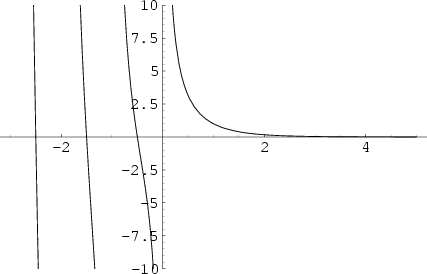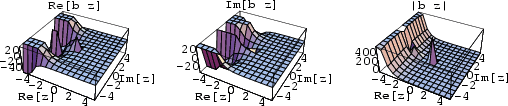The central beta function is defined by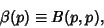(1)

where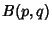is the Beta Function. It satisfies the identities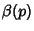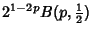(2)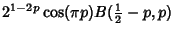(3)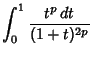(4)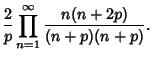(5)

With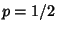, the latter gives the Wallis Formula. When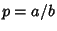,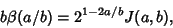(6)

where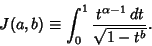(7)

The central beta function satisfies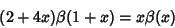(8)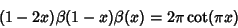(9)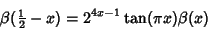(10)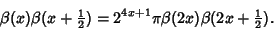(11)

For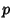an Odd Positive Integer, the central beta function satisfies the identity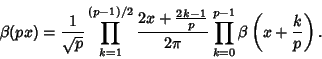(12)

Borwein, J. M. and Zucker, I. J. Elliptic Integral Evaluation of the Gamma Function at Rational Values of Small Denominators.'' IMA J. Numerical Analysis 12, 519-526, 1992.